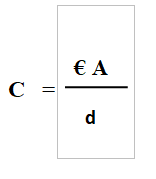# Capacitors:

Before knowing how does a capacitor works, it is essential to know basics of capacitor and what exactly a capacitor is
• Capacitor is component which is used for storing energy in electric charge.
• The capacitance is measured is the unit Farads.
• It is a passive component, so it does not need any electrical power to operate.
• Capacitor can be fixed capacitor, variable capacitor, polarised capacitor.

CONSTRUCTION OF CAPACITOR

The capacitor is made up of two conducting plates, between these two conducting plates dielectric material is used such as paper, glass mica etc. Dielectric simply means insulator which can be polarised if electric field is applied to it.

#### FUNCTION OF CAPACITOR

• How the capacitor gets charged ???

When a capacitor is connected in a circuit upper terminal or top terminal of the capacitor is connected to the positive terminal of the battery and the bottom terminal of the capacitor is connected to negative terminal of the battery. Initially the two conducting plates are neutral. When the voltage is applied to it the electrons from upper plate of capacitor gets attracted towards the positive terminal of the battery and through the negative terminal of the battery the electrons are pushed towards the lower plate of the capacitor. But keep in mind that dielectric material does not allow any flow of electrons through itself. With this process the upper plate of capacitor will get positively charged and the bottom plate of the capacitor will get negatively charged.

• Discharging of capacitor

When a load is connected in that circuit with capacitor and switch is turned ON. Lets consider the load is a bulb. Then the electron will move from bottom plate towards the upper plate or top plate through that load. Because of this transfer of electrons from bottom plate to top plate the bulb starts glowing. When all the electrons are transferred towards top plate then only the whole process will stop and bulb will stop glowing and the capacitor is now discharged, that is both the plates are now neutral again

HOW TO CALCULATE THE CAPACITANCE OF OF CAPACITOR ?Where,

C = Capacitance of the capacitor.

€ = Permittivity of the dielectric material.

A= Area of the conducting plates.

d= distance between two plates.

We can say that more the surface area of the two plates more will be the Capacitance of the capacitor. Same can be said about the permittivity. But as the distance between two conducting plates is increased then the capacitance will be reduced.

## 1 Comment

•